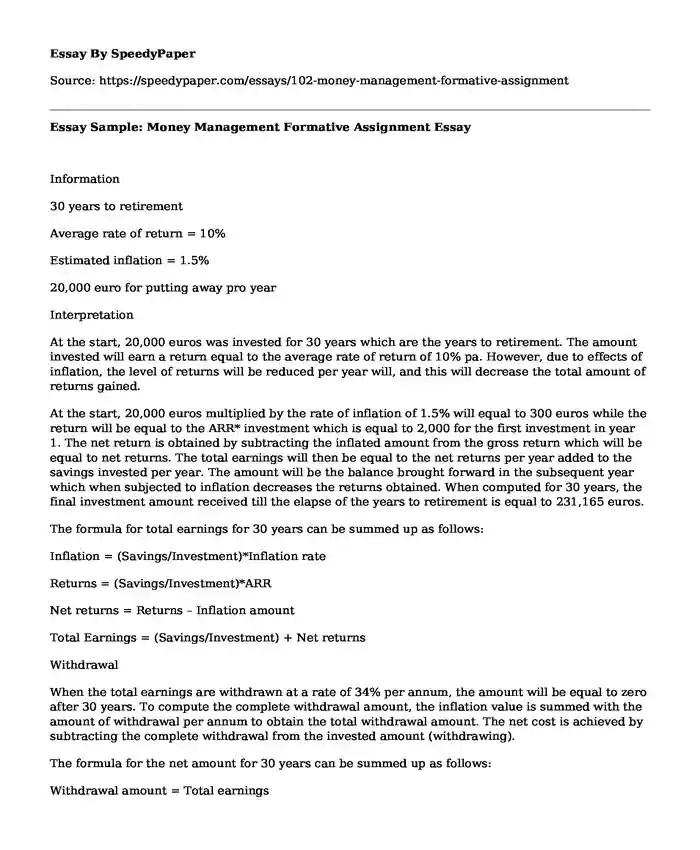# Essay Sample: Money Management Formative Assignment

Published: 2018-03-01Type of paper: Essay Categories: Strategy Finance Money Financial management Pages: 2 Wordcount: 321 words
143 views

InformationIs your time best spent reading someone else’s essay? Get a 100% original essay FROM A CERTIFIED WRITER!

30 years to retirement

Average rate of return = 10%

Estimated inflation = 1.5%

20,000 euro for putting away pro year

Interpretation

At the start, 20,000 euros was invested for 30 years which are the years to retirement. The amount invested will earn a return equal to the average rate of return of 10% pa. However, due to effects of inflation, the level of returns will be reduced per year will, and this will decrease the total amount of returns gained.

At the start, 20,000 euros multiplied by the rate of inflation of 1.5% will equal to 300 euros while the return will be equal to the ARR* investment which is equal to 2,000 for the first investment in year 1. The net return is obtained by subtracting the inflated amount from the gross return which will be equal to net returns. The total earnings will then be equal to the net returns per year added to the savings invested per year. The amount will be the balance brought forward in the subsequent year which when subjected to inflation decreases the returns obtained. When computed for 30 years, the final investment amount received till the elapse of the years to retirement is equal to 231,165 euros.

The formula for total earnings for 30 years can be summed up as follows:

Inflation = (Savings/Investment)*Inflation rate

Returns = (Savings/Investment)*ARR

Net returns = Returns – Inflation amount

Total Earnings = (Savings/Investment) + Net returns

Withdrawal

When the total earnings are withdrawn at a rate of 34% per annum, the amount will be equal to zero after 30 years. To compute the complete withdrawal amount, the inflation value is summed with the amount of withdrawal per annum to obtain the total withdrawal amount. The net cost is achieved by subtracting the complete withdrawal from the invested amount (withdrawing).

The formula for the net amount for 30 years can be summed up as follows:

Withdrawal amount = Total earnings

Inflation = (Withdrawal amount)*Inflation rate

Amount = (Withdrawal amount)*withdrawal rate

Total withdrawal = Amount + inflation amount

Net Amount = Withdrawal amount – total withdrawal

Essay Sample: Money Management Formative Assignment. (2018, Mar 01). Retrieved from https://speedypaper.com/essays/102-money-management-formative-assignment

Request Removal

If you are the original author of this essay and no longer wish to have it published on the SpeedyPaper website, please click below to request its removal:

Liked this essay sample but need an original one?

Hire a professional with VAST experience!